# Cost Accounting MCQs (Set-I) | for FPSC and all other One Paper MCQs Tests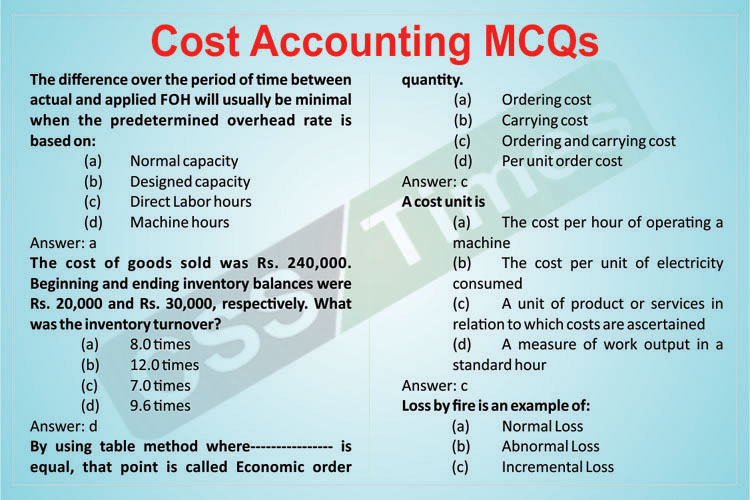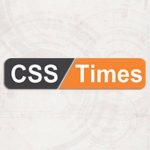Written by

The difference over the period of time between actual and applied FOH will usually be minimal when the predetermined overhead rate is based on:
(a) Normal capacity
(b) Designed capacity
(c) Direct Labor hours
(d) Machine hours

The cost of goods sold was Rs. 240,000. Beginning and ending inventory balances were Rs. 20,000 and Rs. 30,000, respectively. What was the inventory turnover?
(a) 8.0 times
(b) 12.0 times
(c) 7.0 times
(d) 9.6 times

By using table method where—————- is equal, that point is called Economic order quantity.
(a) Ordering cost
(b) Carrying cost
(c) Ordering and carrying cost
(d) Per unit order cost

A cost unit is
(a) The cost per hour of operating a machine
(b) The cost per unit of electricity consumed
(c) A unit of product or services in relation to which costs are ascertained
(d) A measure of work output in a standard hour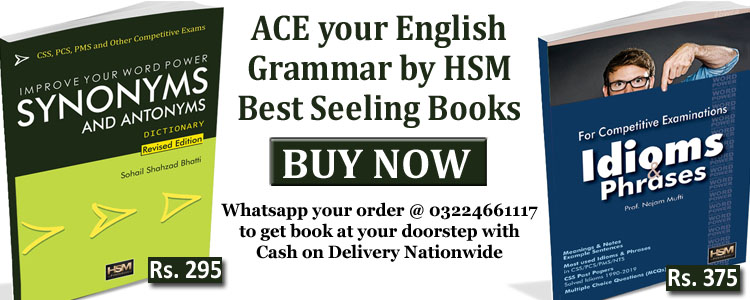Loss by fire is an example of:
(a) Normal Loss
(b) Abnormal Loss
(c) Incremental Loss
(d) Cannot be determined

The main purpose of cost accounting is to
(a) Maximize profits
(b) Help in inventory valuation
(c) Provide information to management for decision making
(d) Aid in the fixation of selling price

Chek Also: PROFESSIONAL TEST MCQs (Set-1) for the Post of Senior Auditor

If, Sales = Rs. 600,000 Markup = 20% of cost What would be the value of Gross profit?
(a) Rs. 200,000
(b) Rs. 100,000
(c) Rs. 580,000
(d) Rs. 740,000

Where the applied FOH cost is less than the actual FOH cost it is:
(a) Unfavorable variance
(b) Favorable variance
(c) Normal variance
(d) Budgeted variance

When the applied cost is lesser than the actual cost it is unfavorable variance. Which of the following is correct?
(a) Units sold=Opening finished goods units + Units produced – Closing finished goods units
(b) Units Sold = Units produced + Closing finished goods units – Opening finished goods units
(c) Units sold = Sales + Average units of finished goods inventory
(d) Units sold = Sales – Average units of finished goods inventory

Which of the following items of expense are to be add in FOH cost
(a) Rent of factory + Head office rent + salaries to factory watchman
(b) Rent of factory + factory lighting bill + Directors salaries
(c) Rent of factory + factory lighting bill + Factory employees salaries
(d) Head office rent + Factory property tax + Factory small tools

If, Gross profit = Rs. 40,000 GP Margin = 20% of sales What will be the value of cost of goods sold?
(a) Rs. 160,000
(b) Rs. 120,000
(c) Rs. 40,000
(d) Rs. 90,000

Taking steps for the fresh purchase of those stocks which have been exhausted and for which requisitions are to be honored in future” is an easy explanation of:
(a) Overstocking
(b) Under stocking
(c) Replenishment of stock
(d) Acquisition of stock

Net Income before Interest and tax is also called:
(a) Operating Income/Profit
(b) Gross Profit
(c) Marginal Income
(d) Other Income

Which of the following is indirect cost?
(a) The depreciation of machinery
(b) The overtime premium incurred at the specific request of a customer
(c) The hire of tools for a specific job
(d) All of the given options

In which of the following center FOH cost NOT incurred
(a) Production Center
(b) Service Center
(c) General Cost Center

Which of the following is considered as basic systems of remunerating labor?
(a) Time rate system
(b) Piece rate system
(d) Both time rate and piece rate system

Net sales = Sales less:
(a) Sales returns
(b) Sales discounts
(c) Sales returns & allowances
(d) Sales returns & allowances and sales discounts

An organistation sold units 4000 and have closing finished goods 3500 units and opening finished goods units were 1000.The quantity of unit produced would be:
(a) 7500 units
(b) 6500 units
(c) 4500 units
(d) 8500 units

Check Also: Accounting Principles & Procedures MCQs (Set-I) for FPSC Senior Auditor Tests

A store ledger card is similar to the ________ .
(a) Stock ledger
(b) Bin card
(c) Material card
(d) Purchase requisition card

Which of the following element must be taken into account while calculating total earnings of a worker under different incentive wage schemes?
(a) Rate per unit
(b) Units of production
(c) Extra time taken by employee to complete the production
(d) Number of workers employed

The journal entry of purchase of stock under periodic inventory system would be?
(a) Inventory to Cash
(b) Cash to Purchases
(c) Purchases to Inventory
(d) None of the given options

Closing work in process Inventory of last year:
(a) Is treated as Opening inventory for current year
(b) Is not carried forward to next year
(c) Become expense in the next year
(d) Charge to Profit & Loss account

The FIFO inventory costing method (when using a perpetual inventory system) assumes that the cost of the earliest units purchased is allocated in which of the following ways?
(a) First to be allocated to the ending inventory
(b) Last to be allocated to the cost of goods sold
(c) Last to be allocated to the ending inventory
(d) First to be allocated to the cost of goods sold

While transporting petrol, a little quantity will be evaporated; such kind of loss is termed as:
(a) Normal Loss
(b) Abnormal Loss
(c) It is incremental loss
(d) It cannot be abnormal loss

The cost of electricity bill of the factory is treated as:
(a) Fixed cost
(b) Variable cost
(c) Step cost
(d) Semi variable cost

A cost centre is
(a) A unit of product or service in relation to which costs are ascertained
(b) An amount of expenidure attributable to an activity
(c) A production or service location, function, activity or item of equipment for which costs are accumulated
(d) A centre for which an individual budget is drawn up

Cost accounting department prepares ___________ that helps the in preparing final accounts.
(a) Cost sheets
(b) Cost of goods sold statement
(c) Cost of production Report
(d) Material requisition form

When FOH is under applied and charged to Net profit , the treatment would be:
(a) Under applied Add net profit
(b) Under applied Less net profit
(c) Under applied Less operating expense
(d) None of the given options

A worker is paid Rs. 0.50 per unit and he produces 18 units in 7 hours. Keeping in view the piece rate system, the total wages of the worker would be:
(a) 18 x 7 x 0.50 = Rs. 63
(b) 18 x 0.50 = Rs. 9
(c) 18 x 7 = Rs. 126
(d) 7 x 0.5 = Rs. 3.5

Weighted average cost per unit is calculated by which of the following formula?
(a) Cost of goods issued/number of units issued
(b) Total cost/total units
(c) Cost of goods manufactured/closing units
(d) Cost of goods sold/total units

Buyer produced 20,000 units and their total factory cost was Rs. 450,000,other cost like property tax on factory building was Rs. 10,000 included in that cost till year ended the cost of per unit would be:
(a) Rs.22.5
(b) Rs.23.5
(c) Rs.24.5
(d) Rs.26.5

Check Also: Principles of Business & Financial Management MCQs

A standard rate is paid to the employee when he completed his job:
(a) In time less than the standard
(b) In standard time
(c) In time more than standard
(d) Both in standard time and more than the standard time

Store incharge after receiving the material as per the goods received note, places the material at its location and makes an entry in_______ .
(a) Bin Card
(b) Store Ledger Card
(c) Stock Ledger
(d) None of these

If opening inventory of material is Rs.20,000 and closing inventory is Rs. 40,000.the Average inventory amount will be:
(a) Rs. 40,000
(b) Rs. 30,000
(c) Rs. 20,000
(d) Rs. 10,000

A company has calculated that volume variance for a given month was unfavorable. This could have been caused by which of the following factors?
(a) The number of rejected units was higher than normal
(b) Machine breakdowns were higher than normal
(c) Delays were experienced in the issuing of material to production
(d) All of the given options

PVC Company has ordering quantity 10,000 units. They have storage capacity 20,000 units, the average inventory would be:
(a) 20,000
(b) 5,000
(c) 10,000
(d) 25,000

If, Gross profit = Rs. 40,000 GP Margin = 25% of sales What will be the value of cost of goods sold?
(a) Rs. 160,000
(b) Rs. 120,000
(c) Rs. 40,000
(d) Cannot be determined

All Indirect cost is charged/record in the head of
(a) Prime cost
(b) FOH cost
(c) Direct labor cost
(d) None of these

Under/Over applied FOH cost can be adjusted in which of the following:
(a) Entire Production
(b) Cost of Goods sold
(c) Net Profit
(d) All of given options

The FIFO inventory costing method (when using a perpetual inventory system) assumes that the cost of the earliest units purchased is allocated in which of the following ways?
(a) First to be allocated to the ending inventory
(b) Last to be allocated to the cost of goods sold
(c) Last to be allocated to the ending inventory
(d) First to be allocated to the cost of goods sold

The danger Level can be calculated?
(a) Average consumption x Lead time to get urgent supplies
(b) Normal consumption x Lead time to get urgent supplies
(c) Maximum consumption x Lead time to get urgent supplies
(d) Minimum consumption x Lead time to get urgent supplies

Chek Also: Journal, Ledger & Cash Book MCQs | for FPSC and other related One Paper MCQs Tests

Which of the following is considered as basic systems of remunerating labor?
(a) Time rate system
(b) Piece rate system
(d) Both time rate and piece rate system

Which of the following is sales force payroll incentive?
(a) Commission
(b) Shift allowance
(c) Over time payment
(d) Bonus

(a) Factory Rent
(b) Property Tax
(c) Salaries of Factory Clerk
(d) All of the given

Which of the following cannot be used as a base for the determination of overhead absorption rate?
(a) Number of units produced
(b) Prime cost
(c) Conversion cost
(d) Discount Allowed

Cost of goods sold Rs. 30, 000, opening Inventory Rs. 9, 000, Closing inventory Rs. 7,800.What was the inventory turnover ratio?
(a) 3.57 times
(b) 3.67 times
(c) 3.85 times
(d) 5.36 times

FOH applied rate of Rs. 5.60 per machine hour. During the year the FOH to Rs.275,000 and 48,000 machine hours were used. Which one of following statement is correct?
(a) Overhead was under-applied by Rs.6,200
(b) Overhead was over-applied by Rs.6,200
(c) Overhead was under-applied by Rs.7,200
(d) Overhead was over-applied by Rs.7,200

Cost accounting concepts include all of the following EXCEPT:
(a) Planning
(b) Controlling
(c) Sharing
(d) Costing

__ are future costs that effect the current management decision.
(a) Sunk Cost
(b) Standard Cost
(c) Relevant Cost
(d) Irrelevant Cost

Which of the following costs is part of the prime cost for manufacturing company?
(a) Cost of transporting raw materials from the suppliers premises
(b) Wages of factory workers engaged in machine maintenance
(c) Depreciation of truck used for deliveries to customers
(d) Cost of indirect production materials

### Buy Best Books for CSS Current Affairs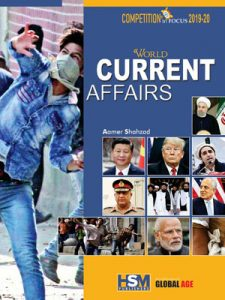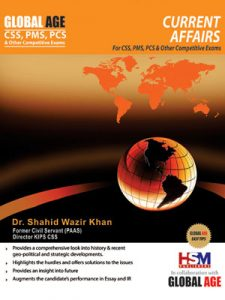Current Affairs by Dr Shahid Wazir Khan Price: Rs. 795 BUY NOW!